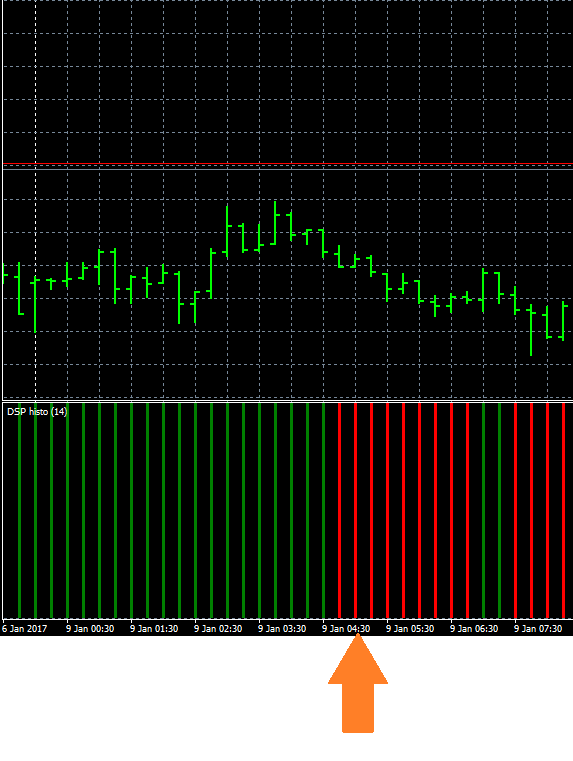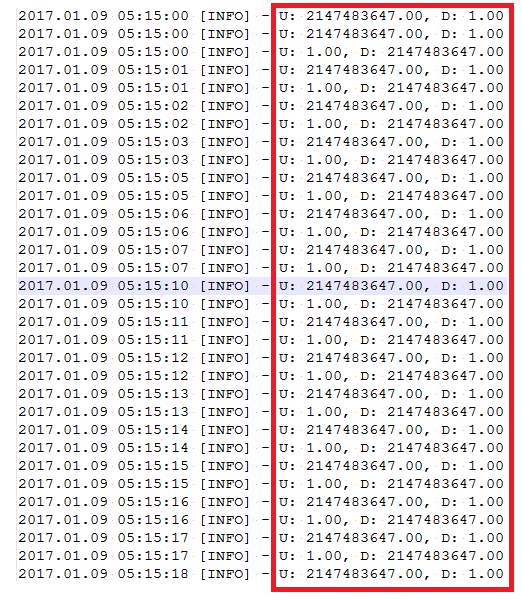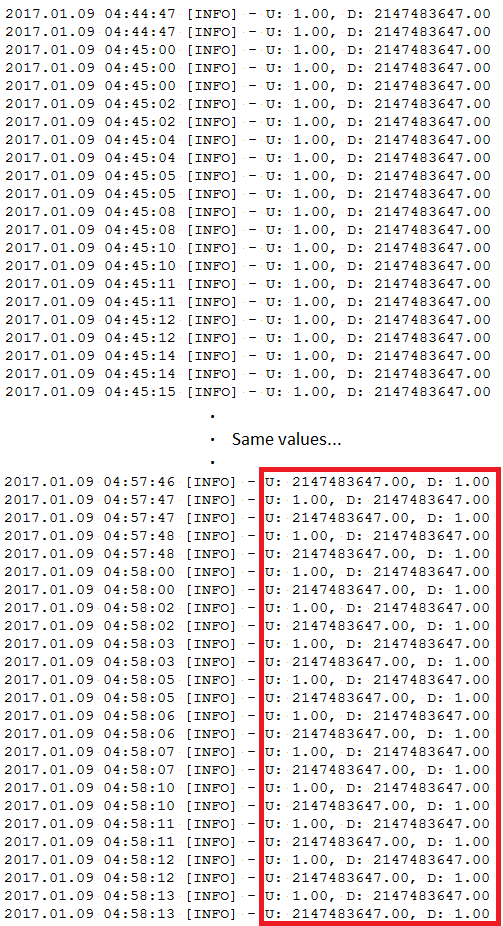Indicator that changes value for each tick, how it is shown?17

Hello,

I was playing around with a downloaded indicator and I noticed that it changes value for each tick. Therefore, within a single bar, it may take different values. I'd like to understand how the indicator is drawn by MetaTrader, since it draws only one bar but the indicator took different values in that bar.

For instance, let's take this part of the indicator between 4:45 and 5:15 (3 bars in total because the graph is M15 period):The indicator is very simple, it has 2 buffers (green bar = "UP", red bar = "DOWN") and each buffer can have only two values, 1 or EMPTY. If "UP" is 1, "DOWN" will be EMPTY, and vice versa. So, if I look at the graph, those 3 red bars means "DOWN". However, if I dump the indicator values for each tick to a file, I see that, in those bars, it continues oscillating between "UP" and "DOWN".

For instance, this is the indicator at 5:15 for each tick, which is shown as "DOWN" bar in the graph:For the bar at 4:45, at the beginning the indicator is "UP" and it starts oscillating only at 4:57, but in the graph it is shown as a "DOWN" bar even if it was "UP" for most of the time:So, my question is: how does MetaTrader "decide" how to draw the indicator for those bars if it hasn't a fixed value?

I hope I explained the problem clearly.

Thanks17

Ok I got it, it draws the last value that the indicator has when the bar is closed